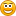# Indicators with alerts/signal - page 17954

Stochastic histogram

Hi i was wondering if anybody has a Stoch Histogram with email/ audio alerts? if not it would really mean a lot to me if someone could code it for me...

Thanks[Deleted]

you can simply change the sample code in MT4 to your indicator. it is only one indicator.54

sample code?

im sorry dont know what were talking about here, really dont know anything about coding so thats why im asking...3203

olivervdm:
Hi i was wondering if anybody has a Stoch Histogram with email/ audio alerts? if not it would really mean a lot to me if someone could code it for me... Thanks

[attach][/attach]

StochHistogram.mq4 2.6 KB 46

StochHistogram.mq4 2.6 KB 475

need sound alarm

Hello all,

Apreciate if somebody here can add sound alert to Support and Resistance (Barry) Indicator. Start sounding when it touches sup or res level

Regards,1199

MoreYummy:
Im trying to add alert on top of the arrow to an indicator, so sound, email alert, with the arrow on the screen.

int init()

{

//---- indicator line

IndicatorBuffers(2);

SetIndexStyle(0,DRAW_ARROW);

SetIndexArrow(0,234);

SetIndexStyle(1,DRAW_ARROW);

SetIndexArrow(1,233);

SetIndexBuffer(0,val1);

SetIndexBuffer(1,val2);

//----

return(0);

}

This is the onlything has something to do with the ARROW. But in the start(), I do not see anything that mention any of these value or anything has arrow, style in it. All there is are simple calculation, and , if, and so on.

Out of idea how it put arrow in the chart, otherwise I can just add it near it and put alert, and email alert on at the same location.

You have to use your buffers val1 & val2, look for the code further down where these 2 values are set, e.g. val1 = something; & val2 = something_else; then you code it something like

if (val1 > val2 ) Alert("some message here"); etc then

if (val1 < val2 ) Alert("some other opposite message here");[Deleted]

increase: thanks for the insight.[Deleted]

This is the original code that I want to add the alert. I tried the "", but it gives syntax error. i cant understand what it is trying to do.

Here are the code in the beginning, I left out the one doing the calculation.

int init()

{

// --------- 暑痧尻蜩痼屐 镥痂钿?潆 镱耱痤屙? 氰闱嚆钼

if (Period1>0) F_Period=MathCeil(Period1*Period()); else F_Period=0;

if (Period2>0) N_Period=MathCeil(Period2*Period()); else N_Period=0;

if (Period3>0) H_Period=MathCeil(Period3*Period()); else H_Period=0;

//---- 吾疣徉螓忄屐 1 狍翦?

if (Period1>0)

{

SetIndexStyle(0,DRAW_ARROW,0,1);

SetIndexArrow(0,Symbol_1_Kod);

SetIndexBuffer(0,FP_BuferUp);

SetIndexEmptyValue(0,0.0);

SetIndexStyle(1,DRAW_ARROW,0,1);

SetIndexArrow(1,Symbol_1_Kod);

SetIndexBuffer(1,FP_BuferDn);

SetIndexEmptyValue(1,0.0);

}

//---- 吾疣徉螓忄屐 2 狍翦?

if (Period2>0)

{

SetIndexStyle(2,DRAW_ARROW,0,2);

SetIndexArrow(2,Symbol_2_Kod);

SetIndexBuffer(2,NP_BuferUp);

SetIndexEmptyValue(2,0.0);

SetIndexStyle(3,DRAW_ARROW,0,2);

SetIndexArrow(3,Symbol_2_Kod);

SetIndexBuffer(3,NP_BuferDn);

SetIndexEmptyValue(3,0.0);

}

//---- 吾疣徉螓忄屐 3 狍翦?

if (Period3>0)

{

SetIndexStyle(4,DRAW_ARROW,0,4);

SetIndexArrow(4,Symbol_3_Kod);

SetIndexBuffer(4,HP_BuferUp);

SetIndexEmptyValue(4,0.0);

SetIndexStyle(5,DRAW_ARROW,0,4);

SetIndexArrow(5,Symbol_3_Kod);

SetIndexBuffer(5,HP_BuferDn);

SetIndexEmptyValue(5,0.0);

}

// 吾疣徉螓忄屐 珥圜屙? 溴忤圉栝 ?泐?

int CDev=0;

int CSt=0;

int Mass[];

int C=0;

if (IntFromStr(Dev_Step_1,C, Mass)==1)

{

Stp1=Mass;

Dev1=Mass;

}

if (IntFromStr(Dev_Step_2,C, Mass)==1)

{

Stp2=Mass;

Dev2=Mass;

}

if (IntFromStr(Dev_Step_3,C, Mass)==1)

{

Stp3=Mass;

Dev3=Mass;

}

return(0);

}

//+------------------------------------------------------------------+

//| Custor indicator deinitialization function |

//+------------------------------------------------------------------+

int deinit()

{

//----

//----

return(0);

}

//+------------------------------------------------------------------+

//| Custom indicator iteration function |

//+------------------------------------------------------------------+

int start()

{

if (Period1>0)

CountZZ(FP_BuferUp,FP_BuferDn,Period1,Dev1,Stp1);

if (Period2>0)

CountZZ(NP_BuferUp,NP_BuferDn,Period2,Dev2,Stp2);

if (Period3>0)

CountZZ(HP_BuferUp,HP_BuferDn,Period3,Dev3,Stp3);

return(0);

}

int CountZZ( double& ExtMapBuffer[], double& ExtMapBuffer2[], int ExtDepth, int ExtDeviation, int ExtBackstep )

{

}

...................

It is a 3 level zz hemafor.mp4, trying to add alert to it.424

I am looking for stoch with alert, the alert should be at the level of 80 and 20. thanks for your help49

jacoba:
I am looking for stoch with alert, the alert should be at the level of 80 and 20. thanks for your help

Hi.